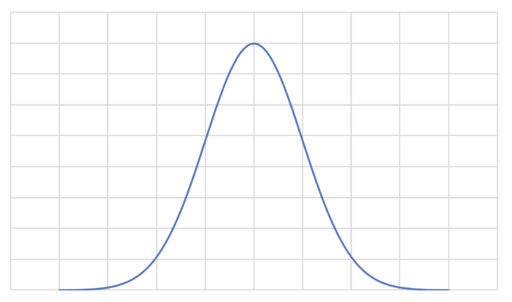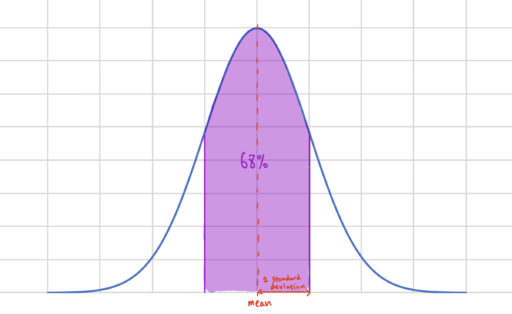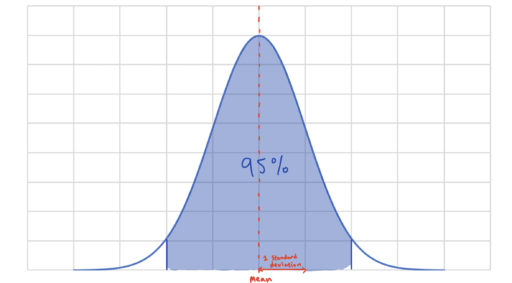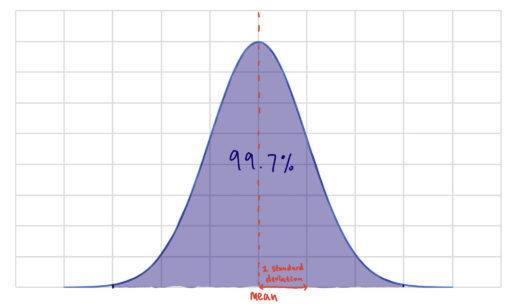The normal distribution is the following curve:and it is important because there is a theorem in statistics (the "Central Limit Theorem") that tells us that if we repeat random experiments of a certain kind, the graph of the different outcomes will look more and more like a normal distribution the more we repeat the experiment. Thus, the outcomes of many random processes (e.g. individual heights), over time, come to be normally distributed.

Crucially, the distribution has the following three properties:

1. It is symmetric about the mean. This means that half of the data is on one side of the mean, and the other half is on the other.
2. The mean of the distribution is equal to the median
3. There are certain facts about how the data is distributed. So:
1. 68% of the data is within 1 standard deviation of the mean
2. 95% of the data is within 2 standard deviations of the mean
3. 99.7% of the data is within 3 standard deviations of the meanNow, we will talk about how to find the standard deviation. There is a formula to do just that:

Suppose we have the following data:. Then, we can find the average:whereis the name commonly used for the average. Then, to find the standard deviation (often denoted), we simply calculate:Sometimes, problems will give you the data and ask you to find the standard deviation. Alternatively, problems may give you the normal distribution and ask you to find the probability of a certain values. Let's look at some examples of each.

Example 1

Over a period of ten days, the value of a bond at the close of trading has had values in accordance with the following table:Find the standard deviation of the bond over these past ten days.

Example 2

Suppose we have a random variable Y which is normally distributed according to a distribution whose mean is 50 and whose standard deviation is 20. What is the probability of the event that Y has a value greater than 90?

Practice Problems

1. Given the following data, find the mean and standard deviation: 12, 23, 75, 54, 34, 24, 13, 53, 24, 3, 33, 10.
2. Given the following data, find the mean and standard deviation: 73, 60, 1021, 584, 12, 807, 1700, 6, 321, 49.
3. The random variable X is distributed normally, with a mean at 43. The probability that X is greater than 50 is .3. What is the probability that X is weakly greater than 36?
4. The random variable Y is normally distributed according to a distribution whose mean is 36 and whose standard deviation is 2. Find the probability that Y is greater than 36.

5. The random variable Y is normally distributed according to a distribution whose mean is 20 and whose standard deviation is 3. Find the probability that Y is less than than 11.